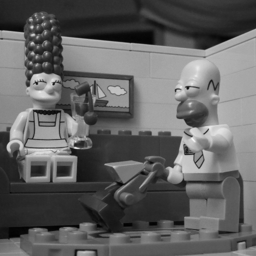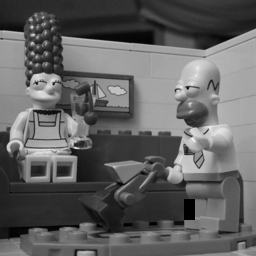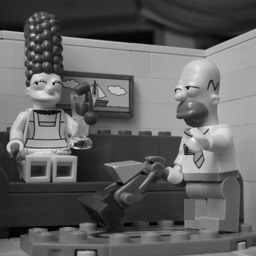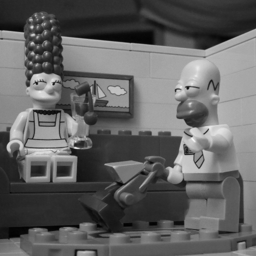# Patch-based Image inpainting

For the following exercices, you need Matlab. The subject of the following exercices is image inpainting.

Many thanks to Alasdair Newson for his help and his Matlab implementation !

## 1 Introduction

In what follows, we describe a simplified version of the inpainting algorithme proposed in

See also the recent paper of Alasdair Newson and coauthors on the same subject

Let $$u$$ be an image defined on $$\Omega$$, and $${\mathcal O}$$ be the occluded region (the hole to inpaint) in $$\Omega$$. We note $${\mathcal D} = \Omega \setminus {\mathcal O}$$ the unoccluded region. The image $$u$$ is known on $${\mathcal D}$$ but unknown on $${\mathcal O}$$.

Let $$P_x$$ be the square patch of size $$(2f+1)^2$$ and centered at $$x$$ in $$u$$ $$P_x = u(x(1)-f:x(1)+f,x(2)-f:x(2)+f).$$ We note $$\tilde{\mathcal D}$$ the set of unoccluded pixels in $$\Omega$$ whose $$(2f+1)\times (2f+1)$$ neighborhoods are also unoccluded.

The idea of Wexler et al. is to minimize the following non-local cost function $E(u,\phi) = \sum_{x \in {\mathcal O}} \| P_x - P_{x+\phi(x)}\|_2^2,$ where $$\phi$$ is a correspondence map between pixels in $$\Omega$$ that must satisfy $$x + \phi(x) \in \tilde{\mathcal D}$$ for all $$x \in \Omega$$. This cost function being non convex, we will use an heuristic approach to minimize it alternatively in $$u$$ and $$\phi$$.

After an initialization, this functional is optimised using the following two steps:

• Matching Given $$u$$, find in $$\tilde{\mathcal D}$$ the nearest neighbour of each patch $$P_x$$ that has pixels in the inpainting domain $${\mathcal O}$$, that is, the map $$\phi(x), \forall x \in \Omega \setminus \tilde{\mathcal D}$$.
• Reconstruction Given the shift map $$\phi$$, attribute a new value $$u(x)$$ to each pixel $$x \in {\mathcal O}$$.

These steps are iterated so as to converge to a satisfactory solution. The process may be seen as an alternated minimisation of the previous cost $$E$$ over the shift map $$\phi$$ and the image content $$u$$.

## 2 Initialization

• Read an image $$u_0$$ and create a small occluded region in $$u_0$$. Image inpainting is computationally quite intensive, so we restrict ourselves to small images (256 x 256) and small holes.
u0 = double(imread('simpson_nb512.png'));
u0 = imresize(u0,[256 256]);                       % resize to 256x256
u = u0; u(mask>0) = 0;
imshow(u,[]);original image u0image u with occluded region in black

• In order to initialize the algorithm, we need a first rough inpainting of $$u$$ in the masked region. Write a code that replaces the values in mask by the mean value of all pixels in the non-occluded area.
u(mask>0) = mean(u(mask==0));
imshow(u,[]);image u with occluded region initialized to the average of the non-occluded pixels

• A better initialization can be obtained by using a TV interpolation in the occluded area (see the assignment on image restoration) or by Poisson editing (see the corresponding assignment).

## 3 Matching

The matching step of the algorithm consists in finding for each pixel $$x$$ in $$\Omega \setminus \tilde{\mathcal D}$$ its nearest neighbor in $$\tilde{\mathcal D}$$. This will define the correspondence map $$\phi$$.

In order to define easily the set $$\tilde{\mathcal D}$$ of unoccluded pixels in $$\Omega$$ whose $$(2f+1)\times (2f+1)$$ neighborhoods are also unoccluded, we start by creating a copy $$uOcc$$ of $$u$$ with very large values inside the mask.

largeScalar = 100000.0;
uOcc = u;


Then we extract all patches in $$u$$ and $$uOcc$$ and rearrange them into columns into two huge matrices thanks to the function im2col of Matlab. In order to obtain matrices with $$n*m$$ columns (where $$[n,m] = size(u)$$), we first expand $$u$$ and $$uOcc$$ by symmetry.

f = 3;    % patch half size


We can now compute the indices of columns in uOccPatch that corresponds to pixels in the set $$\Omega \setminus \tilde{\mathcal D}$$. Those pixels are easily found since some of their neighbors have been set to the very large value largeScalar.

occPatchInds = find(sum(uOccPatch,1)>largeScalar);


The nearest neighbor search is carried out for all patches touching the occlusion mask thanks to the efficient function knnsearch in Matlab. For each patch with a center in $$\Omega \setminus \tilde{\mathcal D}$$, we look for its nearest neighbor inside $$\tilde{\mathcal D}$$. In order to ensure that the nearest neighbor is indeed inside $$\tilde{\mathcal D}$$, the nearest neighbor is found among the patches of uOccPatch. The large values inside the mask in uOccPatch permits to forbid patches in the occlusion to point to themselves.

nnInds = knnsearch(uOccPatch',(uPatch(:,occPatchInds))');


The matching step is almost done at this point. The column vector nnInds contains the indices of correspondents for all pixels in $$\Omega \setminus \tilde{\mathcal D}$$. The length of this vector is the same as the length of occPatchInds.

Finally, we create the vector field $$nnOut(x) = \phi(x)+x$$ from this vector of indices. To this aim, we first create a list of indices poiting to themselves and we put the nearest neighbour indices in the correct place.

nnIndsOut = reshape(1:numel(u),size(u));  %list of indices pointing to themselves
nnIndsOut(occPatchInds) = nnInds;         %put the nearest neighbour indices in the correct place
%convert index to subindices
[nnOutY,nnOutX] = ind2sub(size(u),nnIndsOut);
nnOut(:,:,1) = nnOutX;  nnOut(:,:,2) = nnOutY;

• Write a function nnOut = nearest_neighbour_field(u,mask,f) that implements all the previous operations.
function nnOut = nearest_neighbour_field(u,mask,f)
largeScalar = 100000.0;
uOcc = u;
occPatchInds = find(sum(uOccPatch,1)>largeScalar);
nnInds = knnsearch(uOccPatch',(uPatch(:,occPatchInds))');
nnIndsOut = reshape(1:numel(u),size(u));
nnIndsOut(occPatchInds) = nnInds;
[nnOutY,nnOutX] = ind2sub(size(u),nnIndsOut);
nnOut(:,:,1) = nnOutX;  nnOut(:,:,2) = nnOutY;
end


## 4 Reconstruction

In the reconstruction step, we reconstruct the image $$u$$ from the set of correspondences $$\phi$$ with the following formula $u(x) = \sum_{y \in P_x} u(x+\phi(y)) \frac{w(x,y)}{\sum_{z\in P_x} w(x,z)},$ where $$w(x,y)$$ are well chosen weights. In practice, it is important to give more weight to pixels $$y$$ which are close (or inside) the non-occluded area, so we chose $w(x,y) = e^{- \frac{dist(y, {\mathcal O}^c)^2}{\sigma^2(y)}}.$ The value of $$\sigma^2(y)$$ has an important influence on the number of locations $$y$$ taken into account in the reconstruction. In practice, we choose $$\sigma^2(y)$$ as the max between 1 and the 25th percentile of all the distances $$\{dist(y, {\mathcal O}^c)^2;\;y\in P_x\}$$.

function uOut = reconstruct_image(u,mask,f,nnField)

occInds = find(mask>0);      % indices of occluded area
occDistance = bwdist(~mask); % distance to O^c

%convert nearest neighbour field to relative offset
[gridX,gridY] = meshgrid(1:size(u,2),1:size(u,1));
shiftFieldX = nnField(:,:,1)-gridX;
shiftFieldY = nnField(:,:,2)-gridY;

uOut = u;
for ii=1:numel(occInds)
[i,j] = ind2sub(size(u),occInds(ii));  % coordinates of the ii^th point in the occluded area
%get relative shifts of all the patches covering the current pixel
currNNsY = i + shiftFieldY( (i-f):(i+f),(j-f):(j+f) );
currNNsX = j + shiftFieldX( (i-f):(i+f),(j-f):(j+f) );

%clamp nearest neighbours to the image size (replicating border conditions)
currNNsY = max(min(currNNsY,size(u,1)),1);
currNNsX = max(min(currNNsX,size(u,2)),1);

%get the linear indices of the nearest neighbours
nnInds = sub2ind(size(u),currNNsY(:),currNNsX(:));

patchDist = occDistance((i-f):(i+f),(j-f):(j+f));
patchWeights = exp(-patchDist(:).^2/(max(prctile(patchDist(:).^2,25),1)));
patchWeights = patchWeights/sum(patchWeights);
uOut(i,j) = sum(patchWeights.*u(nnInds));

end
end


## 5 Inpainting

• Initialize the half patch size $$f$$ and the number of iterations $$N$$. You should chose $$f$$ smaller than $$4$$ if you want the computing time to remain reasonable…
f = 3;
N = 40;

• Alternate the two previous steps to inpaint the occluded region in $$u$$.
uInpaint = u;
for ii=1:N
ii                                               %display iteration number
nnField = nearest_neighbour_field(uInpaint,mask,f);    %find nearest neighbours
uInpaint = reconstruct_image(uInpaint,mask,f,nnField); % reconstruct image
end

• Display the result
imshow(uInpaint,[]);image u inpainted with 7x7 patches and 40 iterations

Date:

Org version 7.9.3f with Emacs version 24

Validate XHTML 1.0